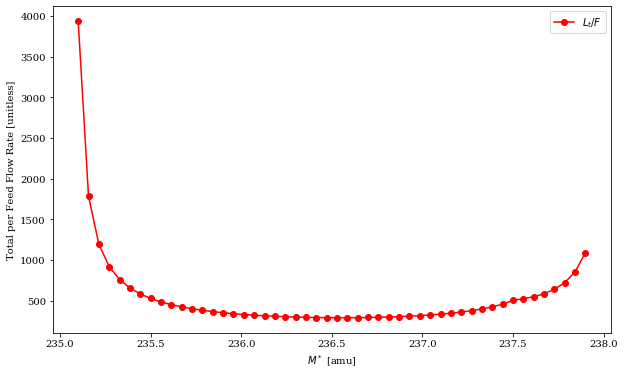# Erichment: M* vs Flowrate¶

Download the full notebook.

mstar_vs_flowrate

# Multicomponent Enrichment¶

This demonstrates how the total normalized flowrate $L_t/F$ in a matched abundance ratio enrichment cascade changes as a function of the key mass $M^*$, an optimization parameter.

In :
import time
import numpy as np
import matplotlib
matplotlib.rc('font', family='serif', size=16)
import matplotlib.pyplot as plt
%matplotlib inline
from pyne import enrichment as enr

NPOINTS = 50
duc = enr.default_uranium_cascade()
mstars = np.linspace(235.1, 237.9, NPOINTS)

In :
start = time.time()
flowrates = []
for mstar in mstars:
duc.Mstar = mstar
casc = enr.solve_symbolic(duc)
flowrates.append(casc.l_t_per_feed)
print("calc took {0:.3g} ms.".format((time.time()-start) * 1e3))

calc took 2.12 ms.

In :
fig, ax = plt.subplots(1, 1, figsize=(10,6))
plt.plot(mstars, flowrates, 'r-o', label='$L_t/F$')
plt.xlabel('$M^*$ [amu]')
plt.ylabel('Total per Feed Flow Rate [unitless]')
lgd = plt.legend(loc=0)In [ ]: